# Introduction

This file contains some examples of the use of the cellMCD function. It reproduces all the figures in the section on real data examples of the report “The cellwise MCD estimator” by J. Raymaekers and P.J. Rousseeuw.

``````library("cellWise")
``````

# Top Gear data

We chose this dataset because both its variables and its cases are fairly easy to interpret. We start by loading the data, select the numerical variables, and rename some of them for nicer visualizations.

``````library(robustHD)
data(TopGear)
dim(TopGear)
``````
``````##  297  32
``````
``````rownames(TopGear) = paste(TopGear[,1],TopGear[,2])
colnames(TopGear)
``````
``````##   "Maker"              "Model"              "Type"
##   "Fuel"               "Price"              "Cylinders"
##   "Displacement"       "DriveWheel"         "BHP"
##  "Torque"             "Acceleration"       "TopSpeed"
##  "MPG"                "Weight"             "Length"
##  "AdjustableSteering" "AlarmSystem"        "Automatic"
##  "Bluetooth"          "ClimateControl"     "CruiseControl"
##  "ElectricSeats"      "Leather"            "ParkingSensors"
##  "PowerSteering"      "SatNav"             "ESP"
##  "Verdict"            "Origin"
``````
``````TopGear = TopGear[c(5,7,9:17)] # objective numerical variables
colnames(TopGear)
``````
``````##   "Price"        "Displacement" "BHP"          "Torque"       "Acceleration"
##   "TopSpeed"     "MPG"          "Weight"       "Length"       "Width"
##  "Height"
``````
``````colnames(TopGear) = c("price", "displacement", "horsepower",
"torque", "acceleration", "top speed",
"miles/gallon", "weight", "length",
"width", "height")
``````

Now we run the checkDataSet routine, to remove any observations with more than half of the variables missing. We additionally rescale the first variable to avoid huge numbers.

``````out = checkDataSet(TopGear, silent = TRUE)
``````
``````##
##  The final data set we will analyze has 295 rows and 11 columns.
##
``````
``````Xorig = out\$remX
dim(Xorig)
``````
``````##  295  11
``````
``````Xorig[,1] = Xorig[,1]/1000
``````
``````##                            price displacement horsepower torque acceleration
## Alfa Romeo Giulietta      21.250         1598        105    236         11.3
## Alfa Romeo MiTo           15.155         1368        105     95         10.7
## Aston Martin Cygnet       30.995         1329         98     92         11.8
## Aston Martin DB9         131.995         5935        517    457          4.6
## Aston Martin DB9 Volante 141.995         5935        517    457          4.6
## Aston Martin V12 Zagato  396.000         5935        510    420          4.2
##                          top speed miles/gallon weight length width height
## Alfa Romeo Giulietta           115           64   1385   4351  1798   1465
## Alfa Romeo MiTo                116           49   1090   4063  1720   1446
## Aston Martin Cygnet            106           56    988   3078  1680   1500
## Aston Martin DB9               183           19   1785   4720    NA   1282
## Aston Martin DB9 Volante       183           19   1890   4720    NA   1282
## Aston Martin V12 Zagato        190           17   1680   4385  1865   1250
``````

Finally, we transform some variables (price, displacement, horsepower, torque, top speed) to get roughly gaussianity in the center:

``````X = Xorig
X[,c(1:4,6)] = log(Xorig[,c(1:4,6)])
``````

There are still some NAs left in the data, most in weight:

``````X = as.matrix(X)
colSums(is.na(X))
``````
``````##        price displacement   horsepower       torque acceleration    top speed
##            0            7            2            2            0            2
## miles/gallon       weight       length        width       height
##           10           31           10           15           10
``````

Now the preprocessing is over, and we can start with analyzing the data further. First we standardize X to Z for marginal outliers. There are indeed quite a few marginal outliers, especially in the MPG variable

``````out = estLocScale(X)
Z = scale(X,center=out\$loc, scale=out\$scale)
cutf = sqrt(qchisq(p=0.99,df=1))
boxplot(Z)
``````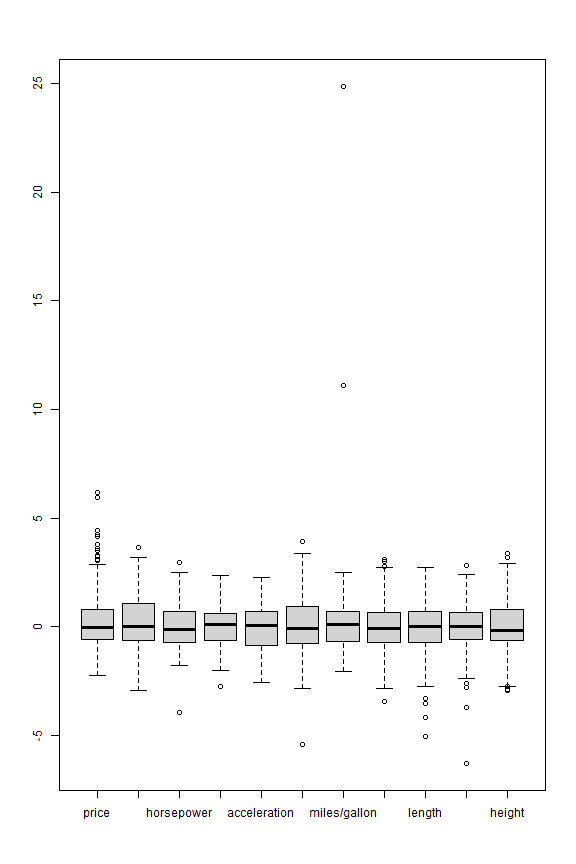As a reference, we can use casewise MCD and inspect the resulting robust distances:

``````out <- robustbase::covMcd(X)
plot(sqrt(out\$mah))
abline(h = sqrt(qchisq(0.99, df = 11)))
``````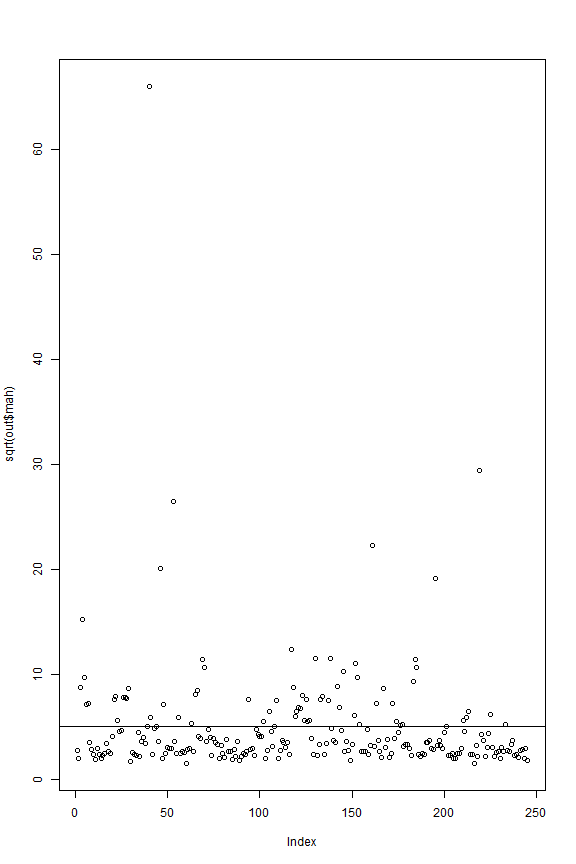Now we run cellMCD:

``````cellout <- cellMCD(X)
``````
``````##
##  The input data has 295 rows and 11 columns.
##
## Objective at step 0 = 7961.68004
## Objective at step 1 = 4962.97691
## Objective at step 2 = 4698.54311
## Objective at step 3 = 4605.28496
## Objective at step 4 = 4546.42427
## Objective at step 5 = 4512.12992
## Objective at step 6 = 4495.17017
## Objective at step 7 = 4489.72644
## Objective at step 8 = 4488.00626
## Objective at step 9 = 4485.83809
## Objective at step 10 = 4485.69376
## Objective at step 11 = 4485.2301
## Objective at step 12 = 4483.45319
## Objective at step 13 = 4481.76062
## Objective at step 14 = 4481.18886
## Objective at step 15 = 4479.10523
## Objective at step 16 = 4476.44302
## Objective at step 17 = 4475.6804
## Objective at step 18 = 4474.97893
## Objective at step 19 = 4474.92711
## Objective at step 20 = 4474.91923
## Objective at step 21 = 4474.91764
## Objective at step 22 = 4474.91726
## Objective at step 23 = 4474.91716
## Percentage of flagged cells per variable:
##        price displacement   horsepower       torque acceleration    top.speed
##        13.56        10.85         5.42         3.73         6.78        12.88
## miles.gallon       weight       length        width       height
##         9.83        18.31         9.83        12.88         5.76
``````

We immediately see 3 huge residuals in MPG, and one in acceleration.

``````Zres = cellout\$Zres
boxplot(Zres)
``````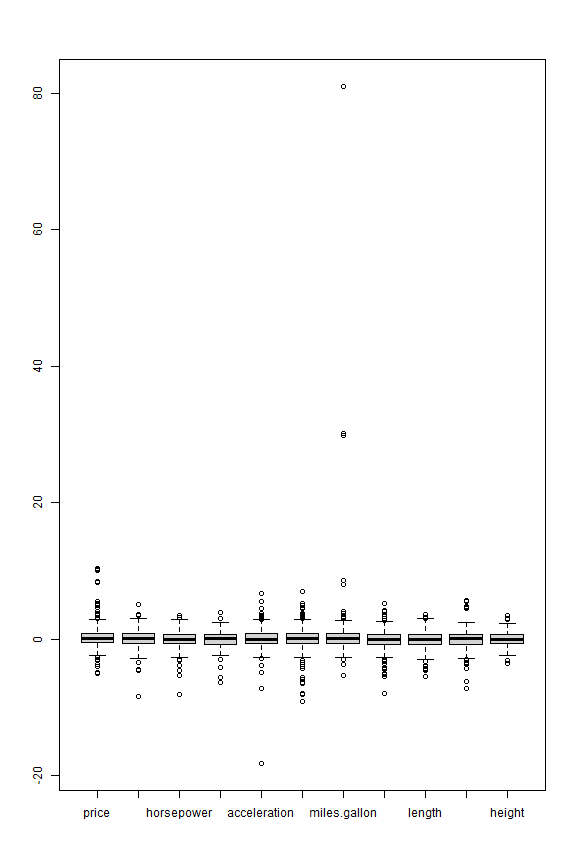``````qqnorm(as.vector(Zres)); abline(0,1)
``````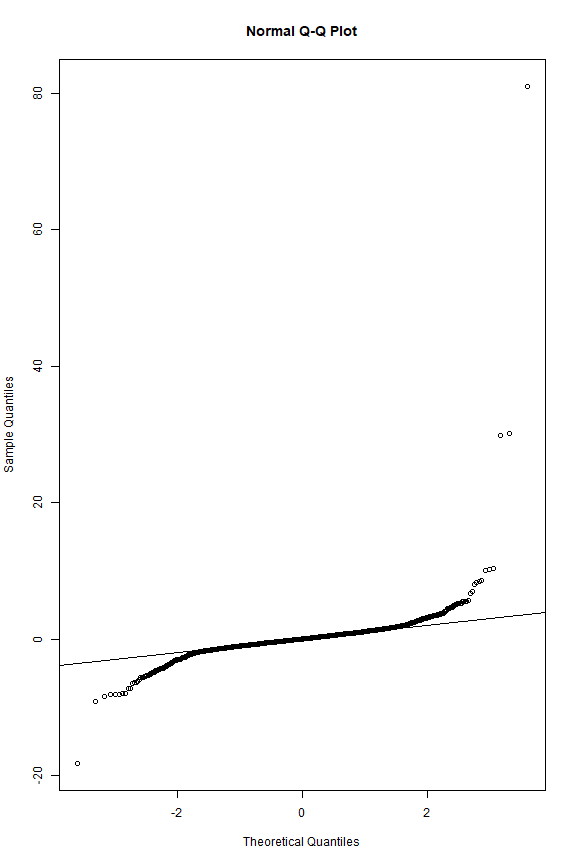How many cells were flagged by cellMCD? In total, 324 cells were flagged. We also see that 151 cards do not have a single flagged cell.

``````W = cellout\$W
sum(as.vector(1-W))
``````
``````##  324
``````
``````rowS = rowSums(1-W)
sum(rowS == 0) # 151 cars do not have a single flagged cell.
``````
``````##  151
``````

Now look at some plots by variable. We start with price:

``````j = 1 # price
# Index plot of Zres:
# (ids = plot.cellMCD(cellout, j, type="index", identify=T)\$ids)
ids = c(6,54,50,195,212,222,221)
Zres[ids,j,drop=F]
``````
``````##                               price
## Aston Martin V12 Zagato   10.192500
## Chevrolet Camaro          -4.881046
## Bugatti Veyron             9.996386
## Pagani Huayra             10.381863
## Proton Satria-Neo         -5.030387
## Rolls-Royce Phantom Coupe  8.225496
## Rolls-Royce Phantom        8.390741
``````
``````plot_cellMCD(cellout, j, type="index", ids=ids)
``````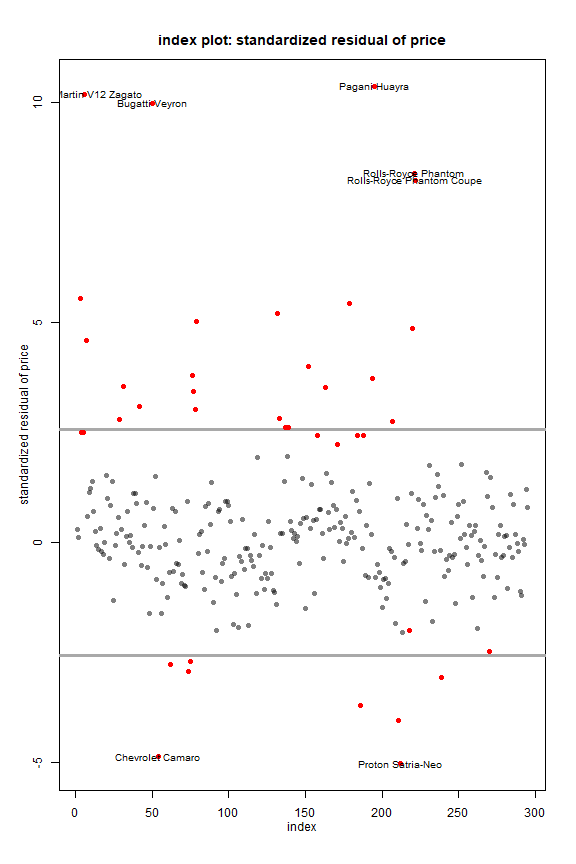We saw that price has quite a few outliers on the right. Camaro and Proton cost less than would be expected based on their other characteristics.

``````# Plot Zres versus X:
plot_cellMCD(cellout, j, type="Zres/X", ids=ids)
``````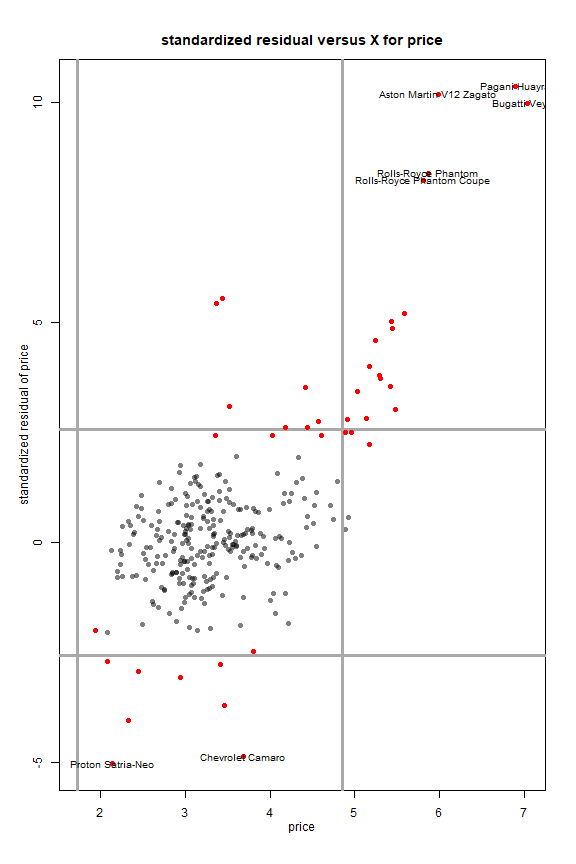``````# Plot Zres versus predicted cell:
plot_cellMCD(cellout, j, type="Zres/pred", ids=ids)
``````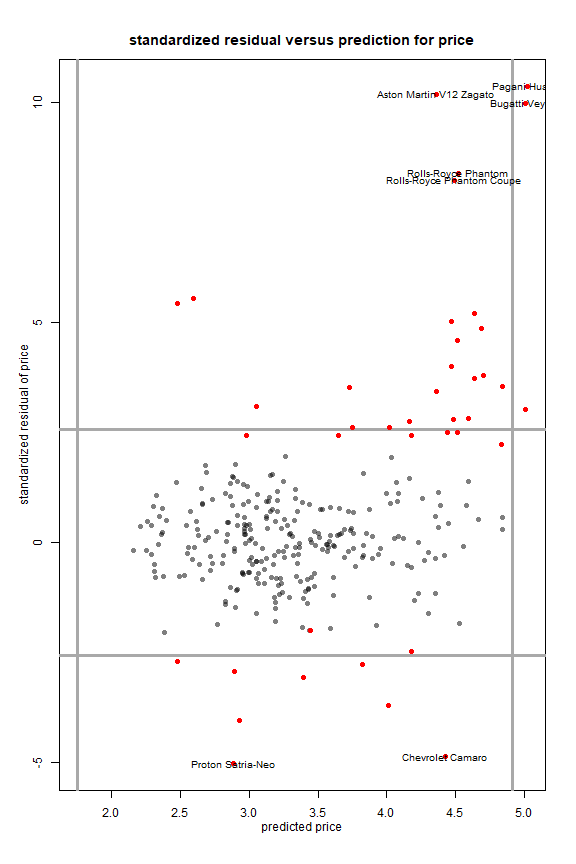``````# Plot observed cell versus predicted cell:
# (ids = plot.cellMCD(cellout, j, type="X/pred", identify=T)\$ids)
ids = c(179,3,212,218,54,6,222,221,50,195)
Xorig[ids,j,drop=F]
``````
``````##                              price
## Mitsubishi i-MiEV           29.045
## Aston Martin Cygnet         30.995
## Proton Satria-Neo            8.495
## Renault Twizy                6.950
## Chevrolet Camaro            39.995
## Aston Martin V12 Zagato    396.000
## Rolls-Royce Phantom Coupe  333.130
## Rolls-Royce Phantom        352.720
## Bugatti Veyron            1139.985
## Pagani Huayra              990.000
``````
``````plot_cellMCD(cellout, j, type="X/pred", vband=T, hband=T, ids=ids)
``````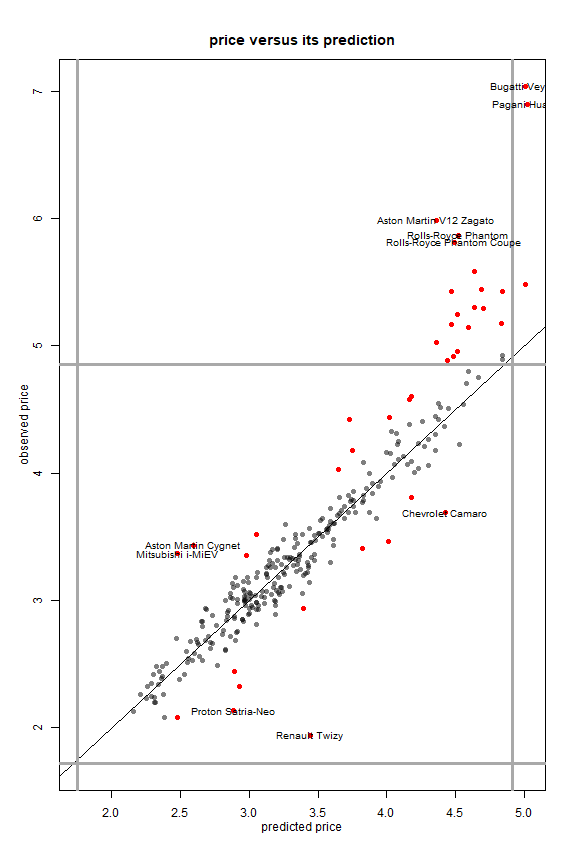The code below reproduces Figure 2 of the paper.

``````###########
## Figure 2
###########

# pdf(file="TopGear_fig2_test.pdf",width=5.2,height=10.4)
par(mfrow=c(2,1)) # (nrows,ncols)
rn = rownames(X) # for object labels
#
######### 1. HP
par(mar = c(3.6, 3.5, 2.8, 1.0))
j = 3
ids = c(52,59,70,218)
labs = rn[ids]; labs = "Caterham"
xvals = c(52, 59, 70, 218)
yvals = c(-5.70, -7.70, -4.20, -4.35)
plot_cellMCD(cellout, j, type="index")
text(x = xvals, y = yvals, labels = labs, cex = 0.9)
#
######### 2. Length
par(mar = c(3.6, 3.5, 2.8, 1.0))
#
j = 9
ids = c(3,50,51,119,144,195,218,232,249)
labs = rn[ids]
labs = "Aston Cygnet"; labs = "Caterham"
xvals = c(2560, 5050, 3100, 5252, 4245,
4605, 2360, 2700, 3330)
yvals = c(-4.11, -3.88, -3.60, 3.87, -4.30,
-5.00, -5.00, -5.90, -4.85)
plot_cellMCD(cellout, j, type = "Zres/X",
main = "standardized residual versus X for length", xlab = "length (mm)", xlim = c(1970, 6000),  ylim = c(-6, 4.5)) #,ids=ids)
text(x = xvals, y = yvals, labels = labs, cex = 0.9)
``````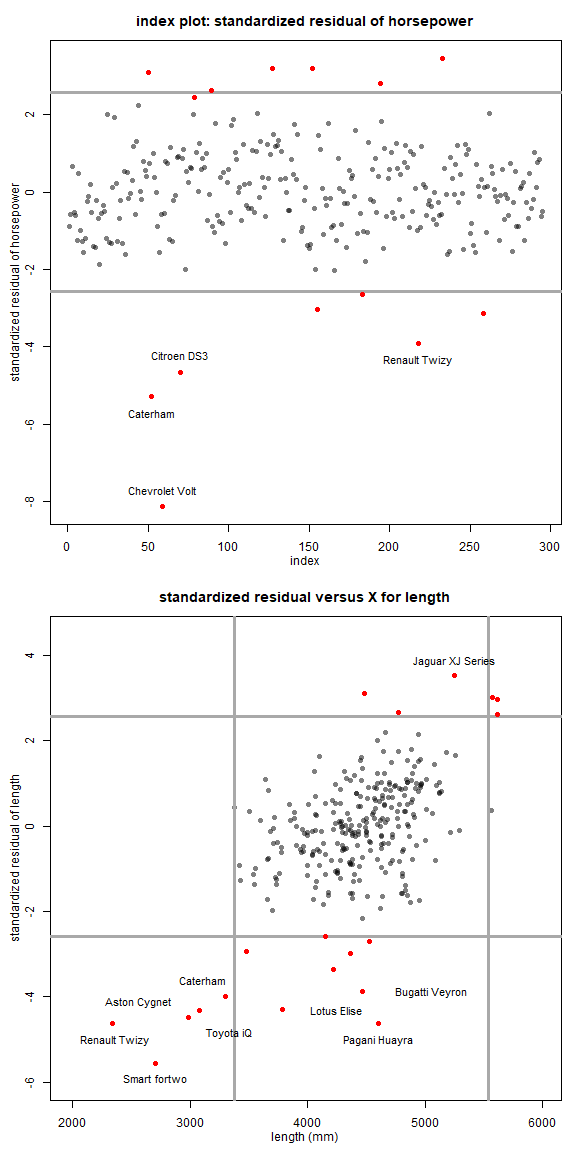``````# dev.off()
``````

Now we reproduce Figure 3.

``````# pdf(file="TopGear_fig3_test.pdf",width=5.2,height=10.4)
par(mfrow=c(2,1))
rn = rownames(X) # for object labels
#
######### 1. weight
par(mar = c(3.6, 3.5, 2.8, 1.0))
j = 8
ids = c(29,51,144,163,183,197,195)
pred = cellout\$preds
labs = rn[ids]
labs = "Caterham"
labs = "Mercedes-Benz G"
xvals = c(1490, 1000, 1890, 1575, 1757,  810, 2250)
yvals = c(5.22,-4.70,-4.73, 4.19,-5.65,-6.05,-7.60)
plot_cellMCD(cellout, j, type="Zres/pred", # vband=F,
xlab = "predicted weight (kg)") # , ids=ids)
text(x = xvals, y = yvals, labels = labs, cex = 0.9)
#
######### 2. top speed
par(mar = c(3.6, 3.5, 2.8, 1.0))
j = 6
ids = c(50,42,52,79,195,218,219,232,258)
labs = rn[ids]
labs = "Caterham"
xvals = c(5.145, 4.937, 5.083, 5.065, 5.076, 4.90, 4.75, 4.63, 5.038)
yvals = c(5.53, 4.53, 4.72, 5.345, 5.44, 3.97, 4.43, 4.30, 4.61)
plot_cellMCD(cellout, j, type="X/pred", vband=F, vlines=F,
xlab="predicted top speed (transformed)")#, ids=ids)
text(x = xvals, y = yvals, labels = labs, cex = 0.9)
``````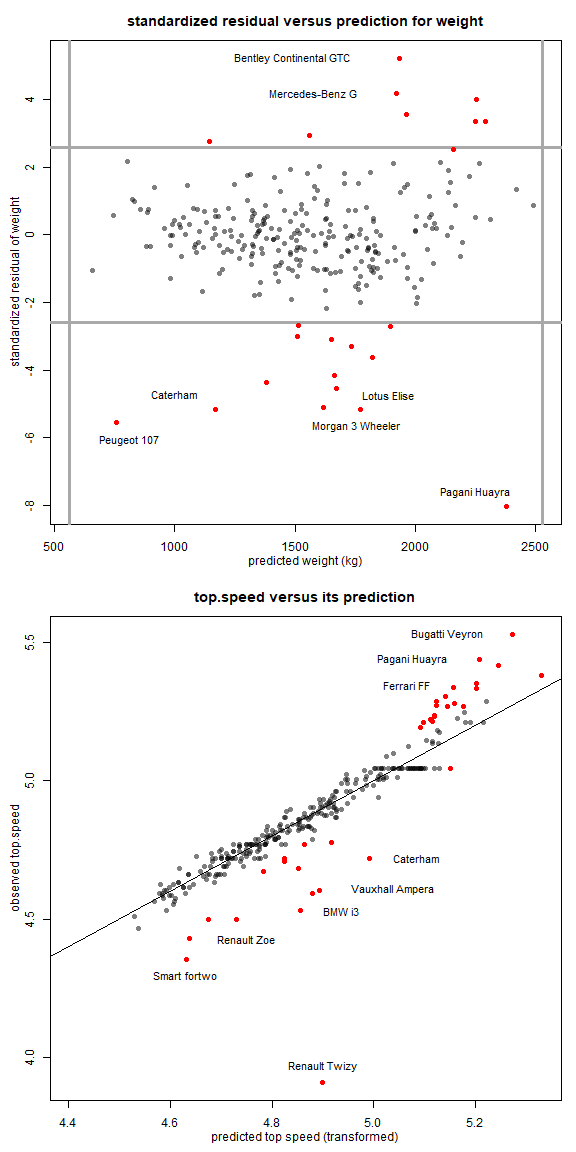``````# dev.off()
``````

Finally, we create Figure 4.

``````############
### Figure 4
############

# pdf(file="TopGear_fig4_test.pdf",width=5.2,height=10.4)
par(mfrow=c(2,1)) # (nrows,ncols)
rn = rownames(X) # for object labels
#
######### 1. MPG versus torque
par(mar = c(3.6, 3.5, 2.8, 1.0))
ids=c(42,258,50)
labs = rn[ids]
xvals = c(5.56, 6.28, 7.27)
yvals = c(470, 235, -16)
plot_cellMCD(cellout, type = "bivariate", horizvar = 4,
vertivar = 7, ids = ids,
xlim=c(3.5,7.7), ylim = c(-27,480),
main = "miles/gallon versus torque",
xlab = "torque (transformed)",
ylab = "miles/gallon",
opacity=0.5, labelpoints=F)
text(x = xvals, y = yvals, labels = labs, cex = 0.9)
#
######### 2. Width versus acceleration
par(mar = c(3.6, 3.5, 2.8, 1.0))
ids = c(218,144,233,51,136,179)
labs = rn[ids]
labs = "Ssangyong" # name was too long for plot
labs = "Caterham"
labs = "Land Rover"
xvals = c(0.0,  -3.0, -3.23,  0.5, 14.2, 15.9)
yvals = c(1200, 1850, 1915, 1688, 2039, 1445)
plot_cellMCD(cellout,  type = "bivariate", horizvar=5,
vertivar=10, ids=ids,
xlab = "acceleration (seconds to 62 mph)",
ylab = "width (mm)", xlim = c(-6.1,20),
ylim = c(1200, 2150),
opacity=0.5, labelpoints=F)
text(x = xvals, y = yvals, labels = labs, cex = 0.9)
``````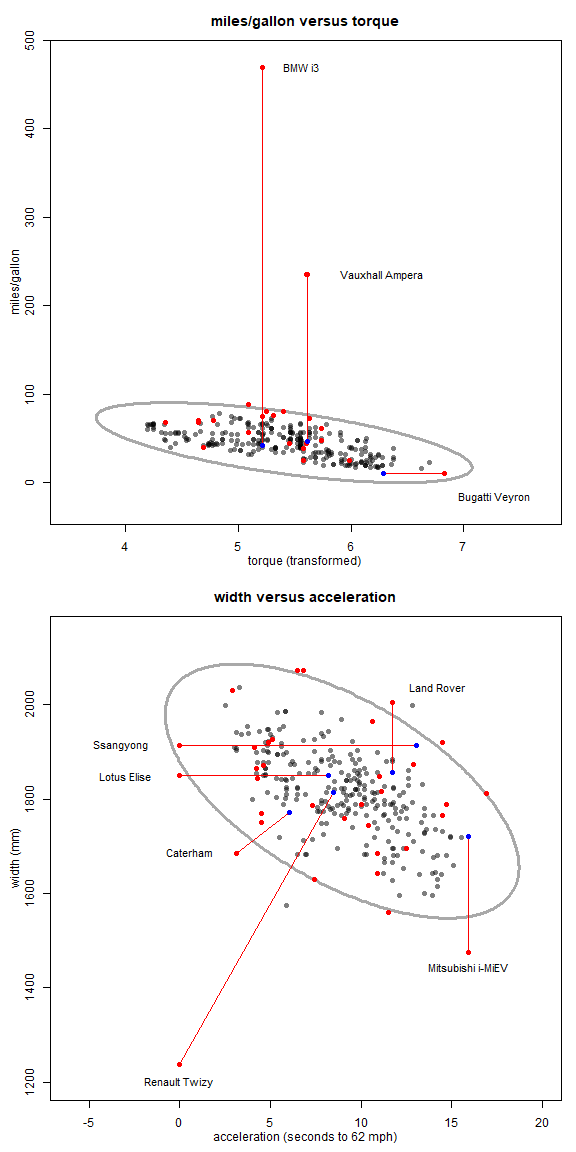``````# dev.off()

par(mfrow=c(1,1))
``````

# Comparison of cellMCD with covMCD

In the following, we compare covMCD and cellMCD on benchmark datasets with casewise outliers. Since some datasets have skewed variables, we always run X = transfo(X)\$Zt before both estimators. We will stop if some variables have 25% or more outliers, as in telef. The easiest way to compare both fits is to plot(rd,cd) and report cor(rd,cd). As said in the report, we require n/d >= 5.

The robustbase library contains most of the datasets as well as the covMCD function.

``````library(robustbase)
``````

## Aircraft data without response (n=23, d=4)

In this dataset we obtain a very high correlation (over 95%) between the robust distances based on covMCD and cellMCD.

``````data(aircraft)
X      <- as.matrix(aircraft)[, 1:4]
pairs(X)
``````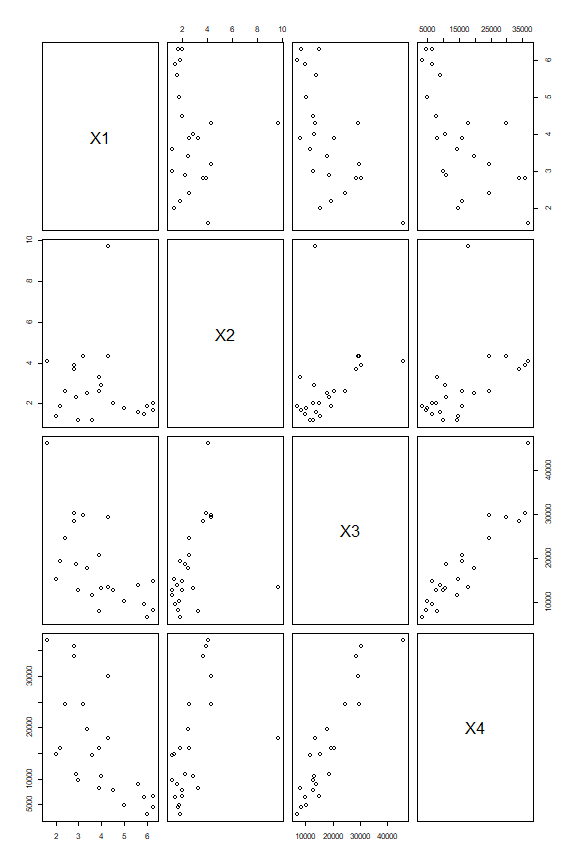``````X      <- transfo(X)\$Zt
``````
``````##
##  The input data has 23 rows and 4 columns.
``````
``````rowout <- robustbase::covMcd(X)
rd     <- sqrt(mahalanobis(X, center = rowout\$center, cov = rowout\$cov))
cutoff <- sqrt(qchisq(0.975,ncol(X)))
sum(rd > cutoff)
``````
``````##  2
``````
``````cellout <- cellMCD(X)
``````
``````##
##  The input data has 23 rows and 4 columns.
##
## Objective at step 0 = 275.20761
## Objective at step 1 = 162.3297
## Objective at step 2 = 145.82557
## Objective at step 3 = 145.03773
## Objective at step 4 = 144.95049
## Objective at step 5 = 144.942
## Objective at step 6 = 144.94115
## Objective at step 7 = 144.94106
## Objective at step 8 = 144.94104
## Percentage of flagged cells per variable:
##    X1    X2    X3    X4
##  8.70 17.39 13.04  8.70
``````
``````cd <- sqrt(mahalanobis(X, center = cellout\$mu, cov = cellout\$S))
plot(rd, cd)
``````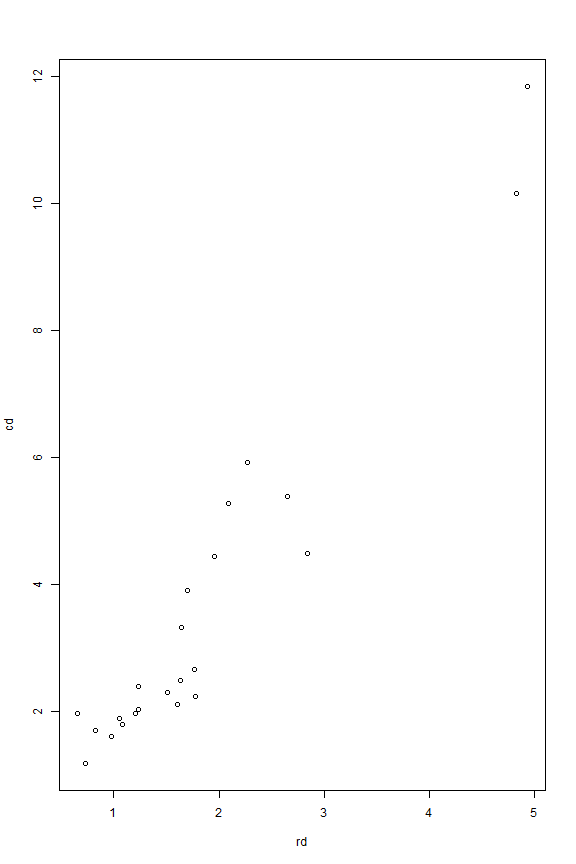``````round(cor(rd, cd),3)
``````
``````##  0.961
``````

## Aircraft with response (n=23, d=5)

When we add the response to the Aircraft dataset, we have n/d < 5. Therefore, the data is no longer suitable for cellMCD.

``````data(aircraft)
X <- as.matrix(aircraft)
nrow(X) / ncol(X)
``````
``````##  4.6
``````

There is also another issue. The response variable has more than 25% marginal outliers. This leads to an error

``````cellout <- cellMCD(X)
``````
``````##
##  The input data has 23 rows and 5 columns.
##
## At least one variable of X has more than 100*(1-alpha)% = 25%
## of marginal outliers.
## The percentages per variable are:
##    X1    X2    X3    X4     Y
##  0.00  4.35 13.04  4.35 30.43
``````
``````## Error in cellMCD(X): Too many marginal outliers.
``````

The transfo function also warns for this:

``````y <- X[, 5]
y <- transfo(y)\$Zt
``````
``````## Warning in transfo(y): The data has 30.43% of outliers, but
## this function was designed for less than 25% of outliers.
``````
``````##
##  The input data has 23 rows and 1 columns.
``````

## alcohol (n=44, d=7)

Here we obtain a correlation between rd and cd of close to 90%. Note that covMCD has a very small eigenvalue (of the order 1e-7). The cellMCD hits its lower bound on the eigenvalues and is thus regularized.

``````data(alcohol, package = "robustbase")
X <- as.matrix(alcohol)
X <- transfo(X)\$Zt
``````
``````##
##  The input data has 44 rows and 7 columns.
``````
``````rowout <- robustbase::covMcd(X)
eigen(rowout\$cov)\$values
``````
``````##  9.975938e+00 8.080408e-02 1.417821e-02 5.314039e-03 1.173304e-04
##  1.230713e-05 3.738181e-07
``````
``````rd <- sqrt(mahalanobis(X, center = rowout\$center, cov = rowout\$cov))
cutoff <- sqrt(qchisq(0.975, ncol(X)))
sum(rd > cutoff)
``````
``````##  15
``````
``````cellout <- cellMCD(X)
``````
``````##
##  The input data has 44 rows and 7 columns.
##
## Objective at step 0 = -432.55468
## Objective at step 1 = -743.36005
## Objective at step 2 = -793.04463
## Objective at step 3 = -800.9642
## Objective at step 4 = -803.0546
## Objective at step 5 = -803.65749
## Objective at step 6 = -809.33995
## Objective at step 7 = -817.49167
## Objective at step 8 = -819.90753
## Objective at step 9 = -820.66418
## Objective at step 10 = -820.90529
## Objective at step 11 = -820.984
## Objective at step 12 = -821.01007
## Objective at step 13 = -821.01877
## Objective at step 14 = -821.02167
## Percentage of flagged cells per variable:
##           SAG             V         logPC             P            RM
##          2.27          2.27         15.91          2.27          2.27
##          Mass logSolubility
##          2.27         15.91
``````
``````cd <- sqrt(mahalanobis(X, center = cellout\$mu, cov = cellout\$S))
round(cor(rd, cd),3)
``````
``````##  0.861
``````

## Animals2 (n=65, d=2)

The original Animals dataset (n=28) is in package MASS and gives a very similar result. Here there is an almost perfect correlation (> 99%) between the distances based on cellMCD and covMCD.

``````data(Animals2)
X <- as.matrix(log(Animals2))
X <- transfo(X)\$Zt
``````
``````##
##  The input data has 65 rows and 2 columns.
``````
``````rowout <- robustbase::covMcd(X)
rd <- sqrt(mahalanobis(X, center = rowout\$center, cov = rowout\$cov))
cellout <- cellMCD(X)
``````
``````##
##  The input data has 65 rows and 2 columns.
##
## Objective at step 0 = 322.45676
## Objective at step 1 = 200.98045
## Objective at step 2 = 196.14567
## Objective at step 3 = 195.45218
## Objective at step 4 = 195.44518
## Objective at step 5 = 195.44506
## Objective at step 6 = 195.44506
## Percentage of flagged cells per variable:
##  body brain
##  9.23  1.54
``````
``````cd <- sqrt(mahalanobis(X, center = cellout\$mu, cov = cellout\$S))
plot(rd, cd)
``````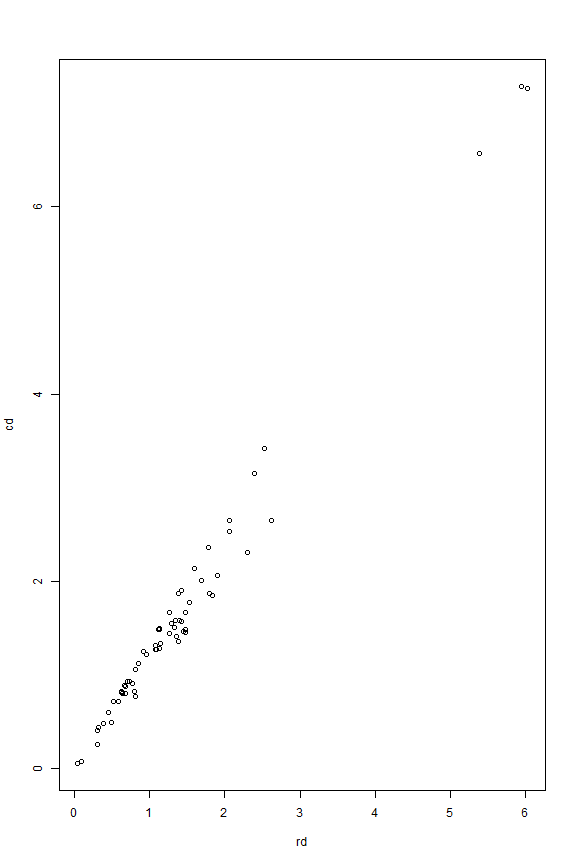``````round(cor(rd, cd), 3)
``````
``````##  0.992
``````

## bushfire (n=38, d=5)

This dataset yields again a very high correlation between rd and cd of over 97%.

``````data(bushfire)
X <- as.matrix(bushfire)
X <- transfo(X)\$Zt
``````
``````##
##  The input data has 38 rows and 5 columns.
``````
``````rowout <- robustbase::covMcd(X)
rd <- sqrt(mahalanobis(X,center=rowout\$center,cov=rowout\$cov))
cutoff <- sqrt(qchisq(0.975, ncol(X)))
sum(rd > cutoff)
``````
``````##  16
``````
``````cellout <- cellMCD(X)
``````
``````##
##  The input data has 38 rows and 5 columns.
##
## Objective at step 0 = 749.59818
## Objective at step 1 = 213.74765
## Objective at step 2 = 145.60671
## Objective at step 3 = 104.88864
## Objective at step 4 = 68.88019
## Objective at step 5 = 45.74439
## Objective at step 6 = 40.73771
## Objective at step 7 = 40.11529
## Objective at step 8 = 37.77965
## Objective at step 9 = 36.79964
## Objective at step 10 = 36.73976
## Objective at step 11 = 36.71931
## Objective at step 12 = 36.71198
## Objective at step 13 = 36.70927
## Objective at step 14 = 36.70825
## Objective at step 15 = 36.70786
## Objective at step 16 = 36.70771
## Objective at step 17 = 36.70765
## Percentage of flagged cells per variable:
##    V1    V2    V3    V4    V5
##  5.26  2.63 10.53 10.53 15.79
``````
``````cd <- sqrt(mahalanobis(X, center = cellout\$mu, cov = cellout\$S))
round(cor(rd, cd), 3)
``````
``````##  0.977
``````

## cloud (n = 19, d = 2)

This small dataset does not really contain outliers. No cells are flagged, and the results of covMCD and cellMCD are very similar

``````data(cloud)
X <- as.matrix(cloud)
X <- transfo(X)\$Zt
``````
``````##
##  The input data has 19 rows and 2 columns.
``````
``````rowout <- robustbase::covMcd(X)
rd <- sqrt(mahalanobis(X, center = rowout\$center, cov = rowout\$cov))
cellout <- cellMCD(X)
``````
``````##
##  The input data has 19 rows and 2 columns.
##
## Objective at step 0 = 5.65676
## Objective at step 1 = 4.10263
## Objective at step 2 = 4.10263
## Percentage of flagged cells per variable:
## Percentage CloudPoint
##          0          0
``````
``````cd <- sqrt(mahalanobis(X,center=cellout\$mu,cov=cellout\$S))
round(cor(rd,cd),3)
``````
``````##  0.97
``````

## delivery without response n=25, d=2

Another example without real outliers. The results of cellMCD and covMCD are very close.

``````data(delivery)
X <- as.matrix(delivery)[, 1:2]
X <- transfo(X)\$Zt
``````
``````##
##  The input data has 25 rows and 2 columns.
``````
``````rowout <- robustbase::covMcd(X)
rd <- sqrt(mahalanobis(X, center = rowout\$center, cov = rowout\$cov))
cutoff <- sqrt(qchisq(0.975, ncol(X)))
sum(rd > cutoff)
``````
``````##  0
``````
``````cellout <- cellMCD(X)
``````
``````##
##  The input data has 25 rows and 2 columns.
##
## Objective at step 0 = 141.79461
## Objective at step 1 = 130.38729
## Objective at step 2 = 130.38729
## Percentage of flagged cells per variable:
##   n.prod distance
##        0        0
``````
``````cd <- sqrt(mahalanobis(X, center = cellout\$mu, cov = cellout\$S))
round(cor(rd, -cd), 3)
``````
``````##  -0.979
``````

## delivery with response n=25, d=3

Now there is a small difference between cellMCD and covMCD. The output of cellMCD is more informative, pointing to the likely source of outlyingness.

``````data(delivery)
X <- as.matrix(delivery)
X <- transfo(X)\$Zt
``````
``````##
##  The input data has 25 rows and 3 columns.
``````
``````rowout <- robustbase::covMcd(X)
rd <- sqrt(mahalanobis(X, center = rowout\$center, cov = rowout\$cov))
cutoff <- sqrt(qchisq(0.975, ncol(X)))
sum(rd > cutoff)
``````
``````##  3
``````
``````cellout <- cellMCD(X)
``````
``````##
##  The input data has 25 rows and 3 columns.
##
## Objective at step 0 = 152.86724
## Objective at step 1 = 136.79294
## Objective at step 2 = 136.72728
## Objective at step 3 = 136.72527
## Objective at step 4 = 136.72521
## Objective at step 5 = 136.7252
## Percentage of flagged cells per variable:
##   n.prod distance  delTime
##        4        0        0
``````
``````cd <- sqrt(mahalanobis(X, center = cellout\$mu, cov = cellout\$S))
round(cor(rd, cd), 3)
``````
``````##  0.851
``````

## exAM (n=12, d=2)

Very similar result between covMCD and cellMCD in terms of location and covariance estimates, and only one suspicious observation is identified. The cellMCD points to the most likely source (i.e. cell) causing the outlyingness of this observation.

``````data(exAM)
X <- as.matrix(exAM)
X <- transfo(X)\$Zt
``````
``````##
##  The input data has 12 rows and 2 columns.
``````
``````rowout <- robustbase::covMcd(X)
rd <- sqrt(mahalanobis(X, center = rowout\$center, cov = rowout\$cov))
cutoff <- sqrt(qchisq(0.975, ncol(X)))
sum(rd > cutoff)
``````
``````##  1
``````
``````cellout <- cellMCD(X)
``````
``````##
##  The input data has 12 rows and 2 columns.
##
## Objective at step 0 = 53.63435
## Objective at step 1 = 51.3864
## Objective at step 2 = 51.37007
## Objective at step 3 = 51.36994
## Objective at step 4 = 51.36994
## Percentage of flagged cells per variable:
##    x    y
## 8.33 0.00
``````
``````cd <- sqrt(mahalanobis(X, center = cellout\$mu, cov = cellout\$S))
round(cor(rd, cd), 3)
``````
``````##  0.983
``````

## hbk without response (n=75, d=3)

Here we find perfect correlation between rd and cd.

``````data(hbk)
X <- as.matrix(hbk)[, 1:3]
X <- transfo(X)\$Zt
``````
``````##
##  The input data has 75 rows and 3 columns.
``````
``````rowout <- robustbase::covMcd(X)
rd <- sqrt(mahalanobis(X, center = rowout\$center, cov = rowout\$cov))
cutoff <- sqrt(qchisq(0.975, ncol(X)))
sum(rd > cutoff)
``````
``````##  14
``````
``````cellout <- cellMCD(X)
``````
``````##
##  The input data has 75 rows and 3 columns.
##
## Objective at step 0 = 755.37911
## Objective at step 1 = 750.55764
## Objective at step 2 = 750.39058
## Objective at step 3 = 750.38204
## Objective at step 4 = 750.38079
## Objective at step 5 = 750.38033
## Objective at step 6 = 750.38014
## Objective at step 7 = 750.38006
## Objective at step 8 = 750.38002
## Objective at step 9 = 750.38001
## Objective at step 10 = 750.38
## Objective at step 11 = 750.38
## Percentage of flagged cells per variable:
##    X1    X2    X3
##  0.00 18.67 18.67
``````
``````cd <- sqrt(mahalanobis(X, center = cellout\$mu, cov = cellout\$S))
round(cor(rd, cd), 3)
``````
``````##  1
``````

## hbk with response (n=75, d=4)

Also here we find perfect correlation between rd and cd.

``````data(hbk)
X <- as.matrix(hbk)
X <- transfo(X)\$Zt
``````
``````##
##  The input data has 75 rows and 4 columns.
``````
``````rowout <- robustbase::covMcd(X)
rd <- sqrt(mahalanobis(X, center = rowout\$center, cov = rowout\$cov))
cutoff <- sqrt(qchisq(0.975, ncol(X)))
sum(rd > cutoff)
``````
``````##  14
``````
``````cellout <- cellMCD(X)
``````
``````##
##  The input data has 75 rows and 4 columns.
##
## Objective at step 0 = 1006.72801
## Objective at step 1 = 1000.56404
## Objective at step 2 = 999.02368
## Objective at step 3 = 998.98142
## Objective at step 4 = 998.97567
## Objective at step 5 = 998.97388
## Objective at step 6 = 998.9732
## Objective at step 7 = 998.97292
## Objective at step 8 = 998.97281
## Objective at step 9 = 998.97276
## Objective at step 10 = 998.97273
## Objective at step 11 = 998.97273
## Objective at step 12 = 998.97272
## Objective at step 13 = 998.97272
## Percentage of flagged cells per variable:
##    X1    X2    X3     Y
##  0.00 18.67 18.67 13.33
``````
``````cd <- sqrt(mahalanobis(X, center = cellout\$mu, cov = cellout\$S))
round(cor(rd, cd), 3)
``````
``````##  1
``````

## kootenay (n=13, d=2)

Perfect correlation between rd and cd.

``````data(kootenay)
X <- as.matrix(kootenay)
X <- transfo(X)\$Zt
``````
``````##
##  The input data has 13 rows and 2 columns.
``````
``````rowout <- robustbase::covMcd(X)
rd <- sqrt(mahalanobis(X, center = rowout\$center, cov = rowout\$cov))
cutoff <- sqrt(qchisq(0.975, ncol(X)))
sum(rd > cutoff)
``````
``````##  1
``````
``````cellout <- cellMCD(X)
``````
``````##
##  The input data has 13 rows and 2 columns.
##
## Objective at step 0 = 43.02753
## Objective at step 1 = 39.64099
## Objective at step 2 = 39.53039
## Objective at step 3 = 39.52422
## Objective at step 4 = 39.52382
## Objective at step 5 = 39.5238
## Objective at step 6 = 39.5238
## Objective at step 7 = 39.5238
## Percentage of flagged cells per variable:
##   Libby Newgate
##    7.69    0.00
``````
``````cd = sqrt(mahalanobis(X, center = cellout\$mu, cov = cellout\$S))
round(cor(rd, cd), 3)
``````
``````##  1
``````

## lactic (n=13, d=2)

This data set is too discrete. It has only 5 unique values for the first variable. This runs into an error:

``````data(lactic)
X <- as.matrix(lactic)
X <- transfo(X)\$Zt
``````
``````##
##  The input data has 20 rows and 2 columns.
##
##  The data contained 1 discrete columns with 5 or fewer values.
##  Their column names are:
##
##  X
##
``````
``````## Error in checkDataSet(X, fracNA = 1, numDiscrete = checkPars\$numDiscrete, :  Only 1 column remains, so we stop.
``````

## milk (n=68, d=8)

Despite substantial numbers of cells flagged in several variables, the correlation between cellMCD and covMCD is over 99%.

``````data(milk)
X <- as.matrix(milk)
X <- transfo(X)\$Zt
``````
``````##
##  The input data has 86 rows and 8 columns.
``````
``````rowout <- robustbase::covMcd(X)
rd <- sqrt(mahalanobis(X, center = rowout\$center, cov = rowout\$cov))
cellout <- cellMCD(X)
``````
``````##
##  The input data has 86 rows and 8 columns.
##
## Objective at step 0 = 2023.97098
## Objective at step 1 = 1281.47891
## Objective at step 2 = 1201.11062
## Objective at step 3 = 1190.51467
## Objective at step 4 = 1180.55133
## Objective at step 5 = 1179.09076
## Objective at step 6 = 1178.91898
## Objective at step 7 = 1178.87506
## Objective at step 8 = 1168.25516
## Objective at step 9 = 1167.04189
## Objective at step 10 = 1166.98988
## Objective at step 11 = 1166.97982
## Objective at step 12 = 1166.97738
## Objective at step 13 = 1166.97672
## Objective at step 14 = 1166.97654
## Objective at step 15 = 1166.97648
## Objective at step 16 = 1166.97646
## Objective at step 17 = 1166.97646
## Percentage of flagged cells per variable:
##    X1    X2    X3    X4    X5    X6    X7    X8
## 11.63  6.98  8.14  2.33 18.60 11.63 10.47  4.65
``````
``````cd <- sqrt(mahalanobis(X, center = cellout\$mu, cov = cellout\$S))
round(cor(rd, cd), 3)
``````
``````##  0.997
``````

## pension (n=13, d=2)

This dataset has more than 25% outliers in its second variable. The transfo function throws an error:

``````data(pension)
X <- as.matrix(pension)
X <- transfo(X)\$Zt
``````
``````## The following variable has 25% or more outliers:
``````
``````## Reserves
##    27.78
``````
``````## Warning in transfo(X): It is recommended not to transform the
## variable Reserves or to do so manually.
``````
``````##
##  The input data has 18 rows and 2 columns.
``````

## phosphor (n=18, d=3)

Another data set with very similar rd and cd.

``````data(phosphor)
X <- as.matrix(phosphor)
X <- transfo(X)\$Zt
``````
``````##
##  The input data has 18 rows and 3 columns.
``````
``````rowout <- robustbase::covMcd(X)
rd <- sqrt(mahalanobis(X, center = rowout\$center, cov = rowout\$cov))
cellout <- cellMCD(X)
``````
``````##
##  The input data has 18 rows and 3 columns.
##
## Objective at step 0 = 193.90576
## Objective at step 1 = 115.826
## Objective at step 2 = 112.507
## Objective at step 3 = 112.42068
## Objective at step 4 = 112.37518
## Objective at step 5 = 112.35076
## Objective at step 6 = 112.33754
## Objective at step 7 = 112.33037
## Objective at step 8 = 112.32647
## Objective at step 9 = 112.32434
## Objective at step 10 = 112.32319
## Objective at step 11 = 112.32255
## Objective at step 12 = 112.32221
## Objective at step 13 = 112.32202
## Objective at step 14 = 112.32192
## Objective at step 15 = 112.32186
## Percentage of flagged cells per variable:
##   inorg organic   plant
##   16.67    0.00   16.67
``````
``````cd <- sqrt(mahalanobis(X, center = cellout\$mu, cov = cellout\$S))
round(cor(rd, cd), 3)
``````
``````##  0.969
``````

## pilot (n=20, d=2)

This dataset has a very strong correlation between the variables, but no outliers. The results of covMCD and cellMCD are quite close.

``````data(pilot)
X <- as.matrix(pilot)
X <- transfo(X)\$Zt
``````
``````##
##  The input data has 20 rows and 2 columns.
``````
``````rowout <- robustbase::covMcd(X)
rd <- sqrt(mahalanobis(X, center = rowout\$center, cov = rowout\$cov))
cutoff <- sqrt(qchisq(0.975, ncol(X)))
sum(rd > cutoff)
``````
``````##  0
``````
``````cellout <- cellMCD(X)
``````
``````##
##  The input data has 20 rows and 2 columns.
##
## Objective at step 0 = -3.05624
## Objective at step 1 = -4.34766
## Objective at step 2 = -4.34766
## Percentage of flagged cells per variable:
## X Y
## 0 0
``````
``````cd <- sqrt(mahalanobis(X, center = cellout\$mu, cov = cellout\$S))
round(cor(rd, cd), 3)
``````
``````##  0.893
``````

Note that when no cells are flagged, the output of cellMCD is the maximum likelihood estimate, that is, the arithmetic mean and (n-1)/n times the classical covariance matrix:

``````cellout\$mu - colMeans(X)
``````
``````##             X             Y
## -4.106072e-18 -2.503383e-17
``````
``````n <- nrow(X)
cellout\$S - (n - 1) * cov(X) / n
``````
``````##               X             Y
## X -3.330669e-16 -1.110223e-16
## Y -1.110223e-16 -3.330669e-16
``````

## radarImage n=1573, d=5

cellMCd and covMCD again yield a perfect correlation between their robust distances. The data is not elliptical, so caution is warranted.

``````data(radarImage)
X <- transfo(X)\$Zt
``````
``````##
##  The input data has 1573 rows and 5 columns.
``````
``````pairs(X)
``````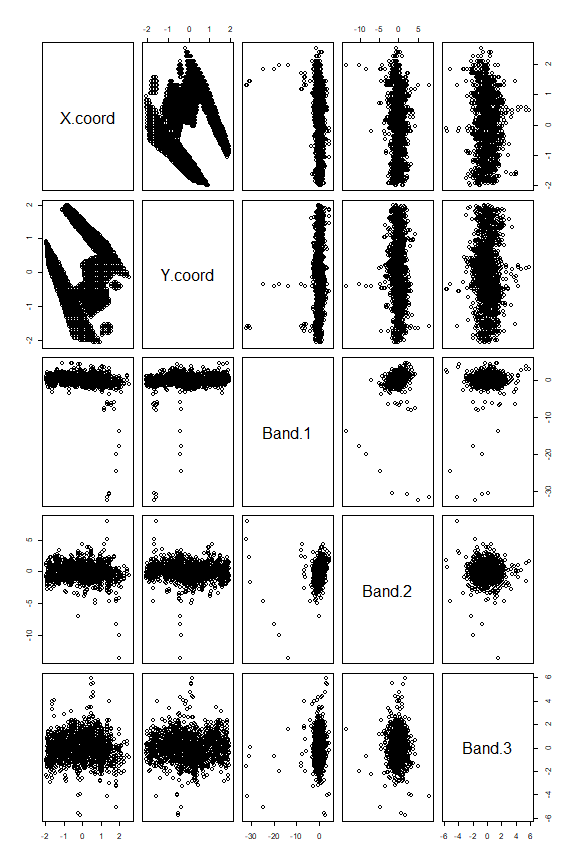``````rowout <- robustbase::covMcd(X)
rd <- sqrt(mahalanobis(X, center = rowout\$center, cov = rowout\$cov))
cutoff <- sqrt(qchisq(0.975,ncol(X)))
sum(rd > cutoff)
``````
``````##  74
``````
``````cellout <- cellMCD(X)
``````
``````##
##  The input data has 1573 rows and 5 columns.
##
## Objective at step 0 = 22208.0531
## Objective at step 1 = 22035.66767
## Objective at step 2 = 22021.84797
## Objective at step 3 = 22020.7372
## Objective at step 4 = 22020.7353
## Objective at step 5 = 22020.73529
## Percentage of flagged cells per variable:
## X.coord Y.coord  Band.1  Band.2  Band.3
##    0.13    0.00    3.56    3.56    2.61
``````
``````cd <- sqrt(mahalanobis(X, center = cellout\$mu, cov = cellout\$S))
round(cor(rd,cd),3)
``````
``````##  1
``````

## Salinity without response n=28, d=3

There are some differences between cellMCD and covMCD on this dataset. Visually, there do not seem to be very extreme outliers. Nevertheless, covMCD discards three observations. cellMCD on the other hand only discards a single cell.

``````data(salinity)
X <- as.matrix(salinity)[, 1:3]
X <- transfo(X)\$Zt
``````
``````##
##  The input data has 28 rows and 3 columns.
``````
``````rowout <- robustbase::covMcd(X)
rd <- sqrt(mahalanobis(X, center = rowout\$center, cov = rowout\$cov))
cutoff <- sqrt(qchisq(0.975, ncol(X)))
sum(rd > cutoff)
``````
``````##  3
``````
``````cellout <- cellMCD(X)
``````
``````##
##  The input data has 28 rows and 3 columns.
##
## Objective at step 0 = 213.68535
## Objective at step 1 = 210.23054
## Objective at step 2 = 210.22597
## Objective at step 3 = 210.22594
## Objective at step 4 = 210.22594
## Percentage of flagged cells per variable:
##   X1   X2   X3
## 0.00 3.57 0.00
``````
``````cd <- sqrt(mahalanobis(X, center = cellout\$mu, cov = cellout\$S))
round(cor(rd,cd),3)
``````
``````##  0.849
``````

## Salinity with response (n=28, d=4)

Now the results between covMCD and cellMCD are a little closer. CellMCD flags two cells, and covMCD flags three rows.

``````data(salinity)
X <- as.matrix(salinity)
X <- transfo(X)\$Zt
``````
``````##
##  The input data has 28 rows and 4 columns.
``````
``````rowout <- robustbase::covMcd(X)
rd <- sqrt(mahalanobis(X, center = rowout\$center, cov = rowout\$cov))
cutoff <- sqrt(qchisq(0.975, ncol(X)))
sum(rd > cutoff)
``````
``````##  3
``````
``````cellout <- cellMCD(X)
``````
``````##
##  The input data has 28 rows and 4 columns.
##
## Objective at step 0 = 240.09105
## Objective at step 1 = 227.11256
## Objective at step 2 = 222.15534
## Objective at step 3 = 216.00885
## Objective at step 4 = 216.00876
## Objective at step 5 = 216.00876
## Percentage of flagged cells per variable:
##   X1   X2   X3    Y
## 3.57 0.00 3.57 0.00
``````
``````cd <- sqrt(mahalanobis(X, center = cellout\$mu, cov = cellout\$S))
round(cor(rd, cd), 3)
``````
``````##  0.887
``````

## starsCYG (n=47, d=2)

On this data the results of covMCD and cellMCD are very close.

``````data(starsCYG)
X <- as.matrix(starsCYG)
X <- transfo(X, nbsteps = 1)\$Zt
``````
``````##
##  The input data has 47 rows and 2 columns.
``````
``````rowout <- robustbase::covMcd(X)
rd <- sqrt(mahalanobis(X, center = rowout\$center, cov = rowout\$cov))
cutoff <- sqrt(qchisq(0.975, ncol(X)))
sum(rd > cutoff)
``````
``````##  5
``````
``````cellout <- cellMCD(X)
``````
``````##
##  The input data has 47 rows and 2 columns.
##
## Objective at step 0 = 252.11346
## Objective at step 1 = 242.86942
## Objective at step 2 = 240.80866
## Objective at step 3 = 240.62807
## Objective at step 4 = 240.60359
## Objective at step 5 = 240.59995
## Objective at step 6 = 240.59939
## Objective at step 7 = 240.59931
## Objective at step 8 = 240.5993
## Objective at step 9 = 240.59929
## Objective at step 10 = 240.59929
## Percentage of flagged cells per variable:
##    log.Te log.light
##     12.77      0.00
``````
``````cd <- sqrt(mahalanobis(X, center = cellout\$mu, cov = cellout\$S))
round(cor(rd,cd),3)
``````
``````##  0.998
``````

## telef n=24, d=2

The transfo function finds too many outliers in Y and gives a warning.

``````data(telef)
X <- as.matrix(telef)
# plot(X)
X <- transfo(X)\$Zt
``````
``````## The following variable has 25% or more outliers:
``````
``````## Calls
## 29.17
``````
``````## Warning in transfo(X): It is recommended not to transform the
## variable Calls or to do so manually.
``````
``````##
##  The input data has 24 rows and 2 columns.
``````

## toxicity (n=38, d=10) does not satisfy n/d > 5

This dataset does not satisy n/d > 5, and there are too many marginal outliers so transfo gives a warning.

``````data(toxicity)
X <- as.matrix(toxicity)
X <- transfo(X, nbsteps = 1)\$Zt
``````
``````## Several variables have 25% or more outliers.
## The percentages per variable are:
``````
``````##   pKa    Ts     P
## 31.58 31.58 44.74
``````
``````## It is recommended not to transform the variables
``````
``````##  "pKa" "Ts"  "P"
``````
``````## or to do so manually.
``````
``````## Warning in transfo(X, nbsteps = 1): There are variables with 25% or more
## outliers.
``````
``````##
##  The input data has 38 rows and 10 columns.
``````

## wood without response n=20, d=5

This data also does not satisfy n/d < 5. cellMCD throws a warning in this case, and caution is warranted.

``````data(wood)
X <- as.matrix(wood)
X <- transfo(X, nbsteps = 1)\$Zt
``````
``````##
##  The input data has 20 rows and 6 columns.
``````
``````cellout <- cellMCD(X)
``````
``````##
##  The input data has 20 rows and 6 columns.
``````
``````## Warning in cellMCD(X): There are fewer than 5 cases per dimension in this data set.
## It is not recommended to run cellMCD on these data.
## Consider reducing the number of variables.
``````
``````##
## Objective at step 0 = 606.67771
## Objective at step 1 = 321.95707
## Objective at step 2 = 285.94055
## Objective at step 3 = 280.74229
## Objective at step 4 = 278.16008
## Objective at step 5 = 276.29299
## Objective at step 6 = 274.7443
## Objective at step 7 = 273.42019
## Objective at step 8 = 272.28833
## Objective at step 9 = 271.33091
## Objective at step 10 = 270.53265
## Objective at step 11 = 269.87752
## Objective at step 12 = 269.34823
## Objective at step 13 = 268.9269
## Objective at step 14 = 268.59604
## Objective at step 15 = 268.33937
## Objective at step 16 = 268.14235
## Objective at step 17 = 267.99251
## Objective at step 18 = 267.87944
## Objective at step 19 = 267.7947
## Objective at step 20 = 267.73154
## Objective at step 21 = 267.68469
## Objective at step 22 = 267.65007
## Objective at step 23 = 267.62456
## Objective at step 24 = 267.60583
## Objective at step 25 = 267.59209
## Objective at step 26 = 267.58203
## Objective at step 27 = 267.57467
## Objective at step 28 = 267.56929
## Objective at step 29 = 267.56537
## Objective at step 30 = 267.5625
## Objective at step 31 = 267.56041
## Objective at step 32 = 267.55888
## Objective at step 33 = 267.55776
## Objective at step 34 = 267.55695
## Objective at step 35 = 267.55635
## Objective at step 36 = 267.55592
## Objective at step 37 = 267.5556
## Objective at step 38 = 267.55536
## Objective at step 39 = 267.55519
## Objective at step 40 = 267.55507
## Objective at step 41 = 267.55497
## Objective at step 42 = 267.55491
## Objective at step 43 = 267.55486
## Objective at step 44 = 267.55482
## Objective at step 45 = 267.55479
## Objective at step 46 = 267.55477
## Objective at step 47 = 267.55476
## Objective at step 48 = 267.55475
## Objective at step 49 = 267.55474
## Objective at step 50 = 267.55473
## Objective at step 51 = 267.55473
## Objective at step 52 = 267.55473
## Objective at step 53 = 267.55472
## Objective at step 54 = 267.55472
## Objective at step 55 = 267.55472
## Percentage of flagged cells per variable:
## x1 x2 x3 x4 x5  y
##  5 15 20 15 10  0
``````